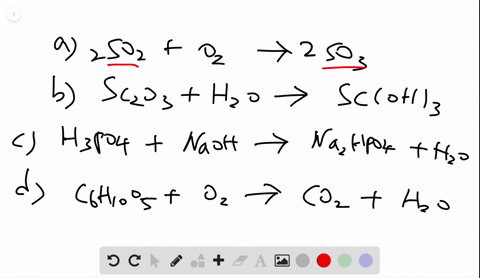Black Friday is Here! Start Your Numerade Subscription for 50% Off!Join Today### Write balanced equations for each of the followin…

04:29University of Toronto
Problem 57

# Write balanced equations for each of the following by inserting the correct coefficients in the blanks:(a) $_{-}\mathrm{Cu}\left(\mathrm{NO}_{3}\right)_{2}(a q)+_{-}\mathrm{KOH}(a q) \longrightarrow _{-}\mathrm{Cu}(\mathrm{OH}_{2}(s)+_{-}\mathrm{KNO}_{3}(a q)$(b) $_{-}\mathrm {BCl}_{3}(g)+_{-}\mathrm{H}_{2} \mathrm{O}(l) \longrightarrow_{-}\mathrm{H}_{3} \mathrm{BO}_{3}(s)+_{-}\mathrm{HCl}(g)$(c) $_{-} \mathrm{CaSiO}_{3}(s)+_{-} \mathrm{HF}(g) \longrightarrow$$_{-}\mathrm{SiF}_{4}(g)+_{-}\mathrm{CaF}_{2}(s)+_{-}\mathrm{H}_{2} \mathrm{O}(l)$(d) $_{-}(\mathrm{CN})_{2}(g)+_{-} \mathrm{H}_{2} \mathrm{O}(l) \longrightarrow_{-}\mathrm{H}_{2} \mathrm{C}_{2} \mathrm{O}_{4}(a q)+_{-} \mathrm{NH}_{3}(g)$

## Discussion

You must be signed in to discuss.